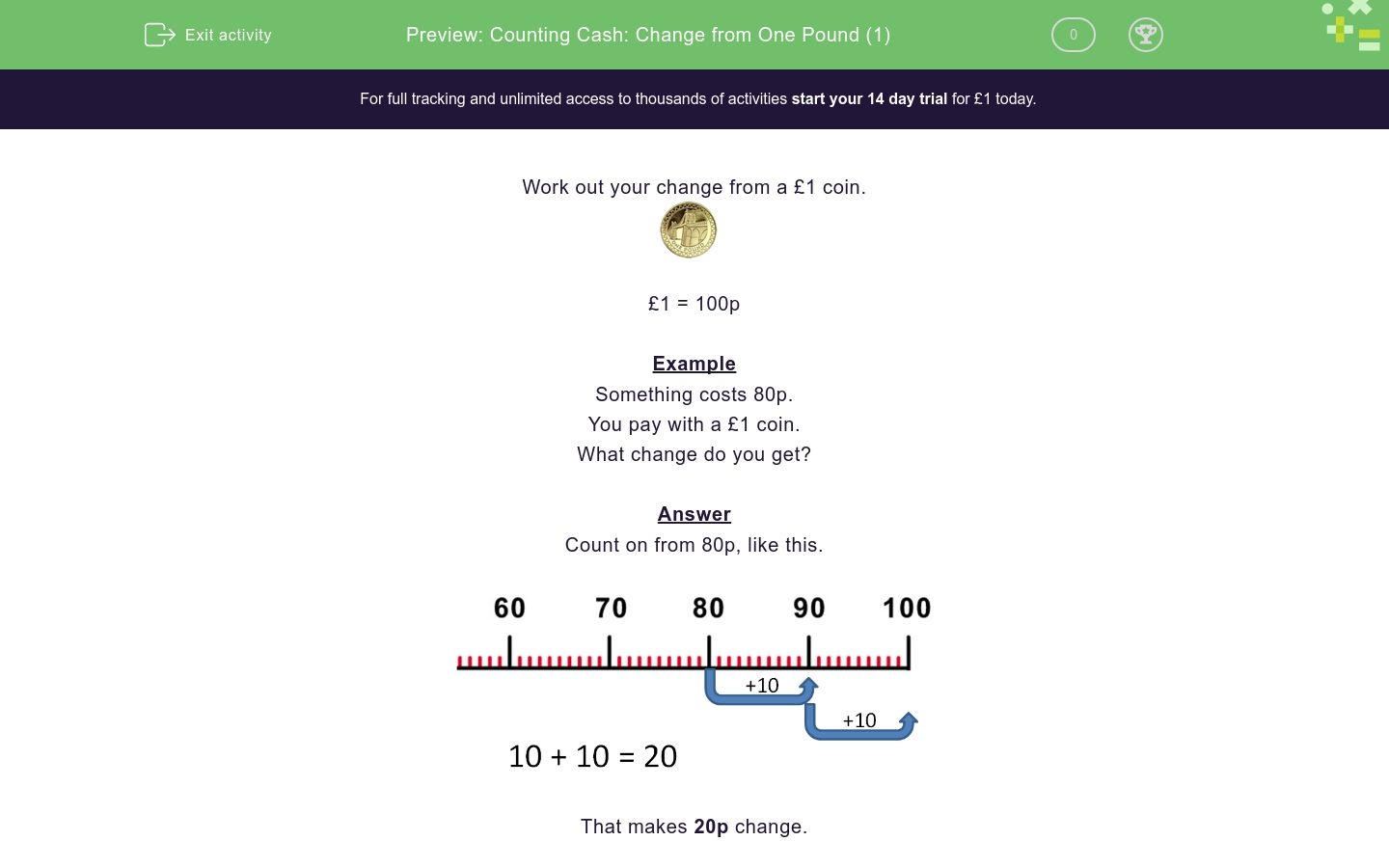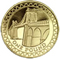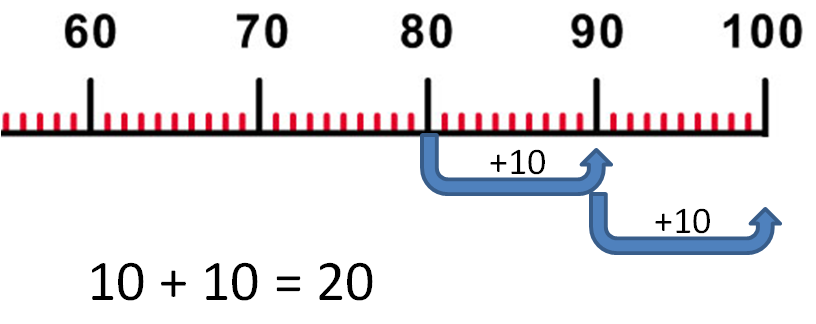# Counting Cash: Change from One Pound (1)

In this worksheet, students calculate the change from a £1 coin for each of the given amounts, using a number line for support.Key stage:  KS 1

Curriculum topic:   Measurement

Difficulty level:### QUESTION 1 of 10

Work out your change from a £1 coin.£1 = 100p

Example

Something costs 80p.

You pay with a £1 coin.

What change do you get?

Count on from 80p, like this.That makes 20p change.

Something costs  50p

You pay with a £1 coin.How much change do you get?

Use the number line to help you by counting on from 50.Something costs 80p

You pay with a £1 coin.How much change do you get?

Use the number line to help you by counting on from 80.You pay for something with a £1 coin.

The thing costs 70p

How much change do you get?

Use the number line to help you by counting on from 70.Something costs 90p

You pay with a £1 coin.How much change do you get?

Use the number line to help you by counting on from 90.Something costs 10p

You pay for it with a £1 coin.How much change do you get?

Use the number line to help you by counting on from 10.Something costs 30p

You pay with a £1 coin.What change will you get?

Use the number line to help you by counting on from 30.You pay for something with a £1 coin.It costs 60p

How much change do you get?

Use the number line to help you by counting on from 60.You pay for something with a £1 coin.It costs 40p

How much change do you get?

Use the number line to help you by counting on from 40.You pay for something with a £1 coin.It costs 99p

How much change do you get?

Use the number line below to help you by counting on from 99.Something costs 85p

You pay with a £1 coin.How much change do you get?

Use the number line below to help you by counting on from 85.• Question 1

Something costs  50p

You pay with a £1 coin.How much change do you get?

Use the number line to help you by counting on from 50.50p
50 p
EDDIE SAYS
Count up from 50p to £1.
Count up in tens.
There are 5 tens.
5 tens = 50
Remember to write a p after the number.
• Question 2

Something costs 80p

You pay with a £1 coin.How much change do you get?

Use the number line to help you by counting on from 80.20p
20 p
EDDIE SAYS
Count in tens from 80p to £1.
That's 2 tens = 20
Remember: write a p after the number.
• Question 3You pay for something with a £1 coin.

The thing costs 70p

How much change do you get?

Use the number line to help you by counting on from 70.30p
30 p
EDDIE SAYS
Count in tens from 70p to 100p (or £1). That's 3 tens = 30
Remember to write the p after the number!
• Question 4

Something costs 90p

You pay with a £1 coin.How much change do you get?

Use the number line to help you by counting on from 90.10p
10 p
EDDIE SAYS
Count up in tens from 90p to £1 (or 100p).
That's 10p.
So you get 10p change.
Remember to write p after the number.
• Question 5

Something costs 10p

You pay for it with a £1 coin.How much change do you get?

Use the number line to help you by counting on from 10.90p
90 p
EDDIE SAYS
Count from 10p to £1 (or 100p).
Count in tens.
There are 9 tens.
• Question 6

Something costs 30p

You pay with a £1 coin.What change will you get?

Use the number line to help you by counting on from 30.70p
70 p
EDDIE SAYS
Count in tens from 30p to £1.
That's 7 tens.
7 tens = 70p. Your change is 70p.
• Question 7

You pay for something with a £1 coin.It costs 60p

How much change do you get?

Use the number line to help you by counting on from 60.40p
40 p
EDDIE SAYS
Count in tens from 60p to £1 (or 100p).
There are 4 tens.
4 tens = 40p.
• Question 8

You pay for something with a £1 coin.It costs 40p

How much change do you get?

Use the number line to help you by counting on from 40.60p
60 p
EDDIE SAYS
Count up in tens.
There are 6 tens.
6 tens = 60p.
• Question 9

You pay for something with a £1 coin.It costs 99p

How much change do you get?

Use the number line below to help you by counting on from 99.1p
1 p
EDDIE SAYS
Count from 99 to 100.
You only have to count 1.
• Question 10

Something costs 85p

You pay with a £1 coin.How much change do you get?

Use the number line below to help you by counting on from 85.15p
15 p
EDDIE SAYS
Count up to 100p (or £1).
(Count from 85.)
Remember to write p after the number.
---- OR ----

Sign up for a £1 trial so you can track and measure your child's progress on this activity.

### What is EdPlace?

We're your National Curriculum aligned online education content provider helping each child succeed in English, maths and science from year 1 to GCSE. With an EdPlace account you’ll be able to track and measure progress, helping each child achieve their best. We build confidence and attainment by personalising each child’s learning at a level that suits them.

Get started